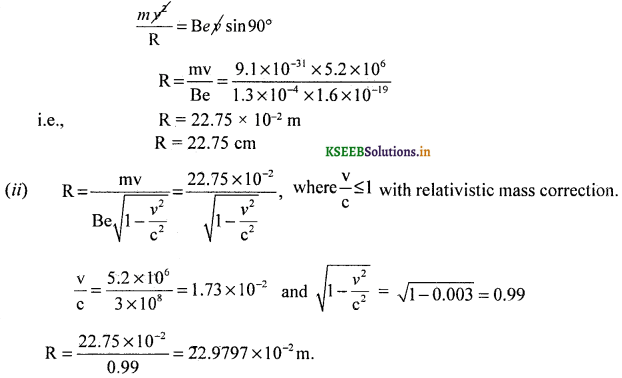# 2nd PUC Physics Question Bank Chapter 11 Dual Nature of Radiation and Matter

Students can Download 2nd PUC Physics Chapter 11 Dual Nature of Radiation and Matter Questions and Answers, Notes Pdf, 2nd PUC Physics Question Bank with Answers helps you to revise the complete Karnataka State Board Syllabus and to clear all their doubts, score well in final exams.

## Karnataka 2nd PUC Physics Question Bank Chapter 11 Dual Nature of Radiation and Matter

### 2nd PUC Physics Dual Nature of Radiation and Matter One Marks Questions and Answers

Question 1.
What is thermionic emission?
The process of electron emission from a heated metal surface is known as thermionic emission.

Question 2.
What is field emission?
The process of electron emission by the application of strong electric / magnetic field at the surface of a metal, is known as field emission.Question 3.
What is secondary emission?
The process of electron emission from the surface of a metal by the bombardment of elementary particles, is known as secondary emission.

Question 4.
What is photo emission?
The process of electron emission from a metal surface when a radiation of suitable frequency is incident on it, is known as photoemission.

Question 5.
What is a photon?
A fixed packet of energy absorbed or emitted by the source of energy is called a photon.

Question 6.
Write the expression for energy of a photon,
Energy of a photon, E = hv, where h is Planck’s constant and v is the frequency of radiation.

Question 7.
What is the value of Planck’s constant?
The value of Planck’s constant, h = 6.625 × 10-34 Js.

Question 8.
What is the speed of photon in free space?
The speed of photon in free space, c = 3 × 108 ms-1.

Question 9.
What is photoelectric effect?
The phenomenon of emission of electrons from the surface of a metal, when a radiation of suitable frequency is made to incident on it, is called photo electric effect.

Question 10.
Who discovered photo electric effect?
Photoelectric effect was discovered by Heinrich Hertz.Question 11.
What is a photoemissive material?
A material which emits electrons when radiation of suitable frequency is incident on it, is called a photoemissive material.

Question 12.
Does photo electric effect confirm the particle nature of light?
Yes, photo electric effect confirms the particle nature of light.

Question 13.
What is threshold frequency?
Threshold frequency is the minimum frequency of the incident radiation below which photo emission cannot take place in the substance.

Question 14.
What is threshold wavelength?
Threshold wavelength is the maximum wavelength of the incident radiation above which no photo emission takes place.

Question 15.
What is stopping potential?
The minimum negative potential of the anode for which all photo electrons are prevented from reaching the anode is called the stopping potential. Stopping potential is directly proportional to the frequency above the threshold frequency.

Question 16.
On what factor does the stopping potential depend for a given photoemitter?
Stopping potential depends on the frequency of the incident radiation.

Question 17.
Write Einstein’s photoelectric equation. (March 2015)
hv = w + $$\frac { 1 }{ 2 }$$mv2, E = hv energy of incident photon.

Question 18.
What is photo electric work function?
The minimum energy which is just enough to liberate electrons from a given surface is called its photo electric work function.Question 19.
What is the principle behind the working of a photoconductive cell?
When light falls on a semi conducting material, its resistance decreases. The resultant increase of current in the circuit is used to operate any auxiliary circuit.

Question 20.
Who introduced the concept of matter waves?
French physicist Louis de-Broglie introduced the concept of matter waves.

Question 21.
Write the de-Broglie’s equation.
The de-Broglie’s equation is λ = $$\frac{h}{m v}$$, where λ is the de Broglie wavelength, m the mass and v the velocity of the particle.

Question 22.
Can wave like properties be associated with macroscopic particles experimentally?
de-Broglie wavelength associated with macroscopic particles is very small and hence can not be detected.

Question 23.
Can the wavelength associated with microscopic particles be detected?
Wavelength associated with microscopic particles like electrons, protons can be observed experimentally.

Question 24.
A particle is accelerated by applying an electric field. How does its de Broglie wave length change?
When a particle is accelerated, its velocity increases and hence its de Broglie wavelength decreases.

Question 25.
How does the de Broglie wavelength of a proton change if its velocity is increased?
As the velocity of a proton increases its de Brogue wavelength decreases.

Question 26.
Give an expression for the energy of photon.
The energy of photon is given by, E = hv.
Where h = Planck’s constant, v = frequency of radiation.
Note : E = hc/λ in terms of wavelength.

Question 27.
Give the expression for energy of photon in terms of eV.
E = $$\frac{h c}{e \lambda}$$ and E = $$\frac{h v}{e}$$ where e = 1.6 × 10-19 C.

Question 28.
Relate linear momentum of photon with wavelength.
Linear momentum of photon p = mc = h/λ i.e., p = h/λQuestion 29.
Give the expression for the moving mass of a photon.
Mass of a photon m = $$\frac{h}{\lambda c}$$ and m = $$\frac{h v}{c^{2}}$$

Question 30.
What the is rest mass energy of a photon?
Zero.

### 2nd PUC Physics Dual Nature of Radiation and Matter Two Marks Questions and Answers

Question 1.
Mention any two types of electron emission. (March 2014)

1. Photo emission and
2. Thermionic emission.

Question 2.
Give any two applications of photo emission.

1. Photo tubes and
2. fire alarms.

Question 3.
Give the expression for (a) mass of a photon and (b) momentum of a photon.
Mass of a photon is m = $$\frac{E}{c^{2}}=\frac{h v}{c^{2}}$$. Momentum of a photon is P = $$\frac{h}{\lambda}$$.

Question 4.
What was Hertz’s observation of photoelectric effect.
Hertz observed that high voltage sparks across the detector loop were enhanced when the emitter plate was illuminated by ultraviolet light from an arc lamp.

Question 5.
What is a photoelectric cell? Mention any two applications of photocells.
Photo cell is a device which converts light energy into electrical energy.
Photocells are used in

1. Automatic fire alarm circuit.
2. Exposure meters.

Question 6.
Represent the variation of PEI v/s intensity of incident light above threshold frequency graphically.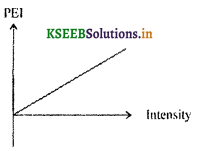Question 7.
Represent the variation of kinetic energy of photoelectrons with respect to frequency graphically.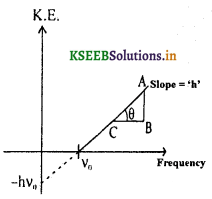K.E. = hv – hv0
slope tan θ = $$\frac{A B}{B C}$$ = h,
-hv0 is the -ve intercept

Question 8.
Represent the variation of PEI v/s anode potential.Question 9.
The threshold wavelength for a metal is 4000 Å. A radiation of wavelength 5000 Å is incident on the metal. Can it cause P.E.E?
No. this is because λ > λ0. The PEE takes place only for λ < λ0.,

Question 10.
What are matter waves? Write de Broglie’s equation.
Waves associated with material particles in motion are called matter waves, de Broglie equation is λ = $$\frac{h}{m v}$$ where λ is the de Broglie wavelength, m is the mass and v is the velocity of the particle.

Question 11.
Draw a neat labelled diagram of experimental arrangement of Davisson – Germer to study electron diffraction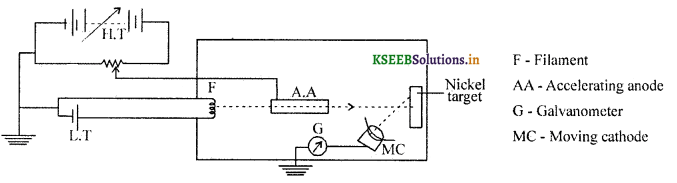Question 12.
Give the expression for wavelength of matter in terms of absolute temperature along with the meaning of the symbols used.
λ = $$\frac{h}{\sqrt{m n k T}}$$
where, m = mass of the particle .
n = number of moles
k = Boltzmann constant
T = absolute temperature
h = Planck’s constant
X = wavelength of matter

Question 13.
Give the expression for the wavelength of a charged particle in terms of accelerating potential and explain the meaning of the symbols used.
The Wavelength, λ = $$\frac{h}{\sqrt{2 q m V}}$$
Where, q = charge on the particle,
m mass of the particle,
V-electric potential in volts.Question 14.
Arrive at the expression for the wavelength of electron in terms of electric potential.
We know that the wavelength associated with the accelerating electron,### 2nd PUC Physics Dual Nature of Radiation and Matter Three Marks Questions and Answers

Question 1.
Mention any three properties of a photon. (March 2014)

1. A photon travels with the speed of light.
2. The rest mass of a photon is zero.
3. Energy of a photon of frequency v is E = hv.

Other properties of a photon:

1. A photon is associated with whole integral spin.
2. A photon has a linear momentum, p = mc.

Question 2.
Distinguish between a photon and a material particle.Question 3.
State the laws of photo emission.
1. The number of photo electrons emitted in a photoelectric process is directly proportional to the intensity the of incident radiation.

2. The maximum kinetic energy of the emitted photo electrons in a photoelectric process is directly proportional to the frequency of the incident radiation.

3. There exists a minimum frequency called threshold frequency below which PEE does not take place in a material.Question 4.
Mention any three applications of photoelectric effect.
Photo electric effect is used in the,

1. reproduction of sound in motion pictures.
2. automatic opening and closing of doors.
3. thickness control of paper in the paper manufacturing.
4. television transmission.

Question 5.
What were Hallwachs’ and Lcnard’s observations on photoelectric effect.
Hallwachs and Lenard observed the ultraviolet radiation were allowed to fall on the emitter plate of an evacuated tube enclosing two metal electrodes, current flows in the circuit. Hallwachs and Lenard also observed that when ultraviolet light made to incident on a emitter plate, no electrons were emitted at all when the frequency of incident light was smaller than a certain minimum value called the threshold frequency.### 2nd PUC Physics Dual Nature of Radiation and Matter Five Marks Questions and Answers

Question 1.
Describe the observed facts of photoelectric effect.

1. Photoe mission is instantaneous
2. For every photo emissive surface there is a minimum frequency of the incident radiation below which there is no photo emission. This minimum frequency is called threshold frequency.
3. For frequency greater than threshold, the strength of the photo electric current is directly proportional to the intensity of the incident radiation.
4. The maximum K.E of the photo electrons increases linearly with the frequency of the incident radiation.
5. There exists a minimum -ve potential called stopping potential for which anode repels the electrons completely.

Question 2.
Give Einstein’s explanation of photoelectric effect. (March 2015)
Einstein assumed that one photoelectron is liberated from a metal surface when one photon of suitable frequency is incident on it. Consider a photon of frequency v and energy hv incident on a photo sensitive metal. The energy of photon is spent in liberating electrons.
1. Since the collision between an electron and a photon is treated as the collision between two particles, the process is instantaneous.

2. If E = hv represents the incident photon energy and ‘w’ is the minimum energy required to just liberate the electron from the surface and K.E. is the kinetic energy of electron then
E = w + K.E.
or hv = hv0 + K.E. → (1) This is known as the P.E. equation.
For K.E. = 0, hv = hv0 = w. Therefore for every metal surface there exists minimum energy of the electron above which electron is liberated. The corresponding frequency is called the threshold frequency and the minimum energy corresponding to v0 is known as the work function.

3. K.E. = $$\frac { 1 }{ 2 }$$mv2
$$\frac { 1 }{ 2 }$$mv2 = hv – hv0
$$\frac { 1 }{ 2 }$$mv2 = h(v – v0)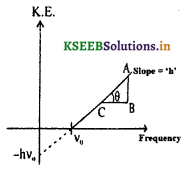∴K.E. and hence velocity of photoelectron depends on the frequency of incident radiation above the threshold frequency (v0) but not on the intensity of incident radiation.

4. For a particular negative potential of the anode, photoelectrons do not reach the anode. Hence P.E. current m = 0. This -ve potential of the anode for which PEI = 0 is known as stopping potential.

5. For v < v0,$$\frac { 1 }{ 2 }$$mv2 = -ve
which is absurd
∴ P.E.E. cannot take place for a frequency less than the threshold frequency.

6. Photo electrons will be ejected in large numbers when intense radiation is incident because intense radiation contains more photons resulting in more interactions.Question 3.
What are matter waves? Derive an expression for the de Broglie wave length.
Waves associated with material particles in motion are called matter waves or de Broglie waves. The energy of a photon is given by E = hv where h is the Planck’s constant and v is the frequency of radiation. If photon is regarded as a particle of mass m, then its energy is given by E = mc2 where c is the velocity of electromagnetic radiation in free space.
E = hv = mc2 …. (1)where p = mc momentum of the photon, de Broglie suggested that equation (2) is applicable to both photons and other material particles. Thus, if a particle of mass m moves with a velocity v, then the deBroglie wave length associated with it isThe above equation is known as de Broglie wave equation.

Question 4.
Explain briefly to demonstrate the wave nature of electron along with a neat labelled diagram of experimental arrangement by Davisson and Germer.
A copious supply of electrons may be obtained by connecting a tungsten filament coated with Barium oxide to a low D.C. voltage.

Electrons are then accelerated by applying a high D.C. supply.
A fine beam is made to fall on the Nickel target.

A moveable collector connected to a galvanometer is moved towards the regions of diffraction. The entire apparatus is kept in vacuum.

For the given angle between the incident and the scattered electron beam, the intensity of beam is measured.

For a known value of anode voltage, wavelength is measured using the formula X = $$\frac{12.27}{\sqrt{\mathrm{V}}}$$Å

It was noticed that a strong peak appeared in the intensity of scattered electrons for an accelerating voltage of 54V at a scattering angle 0 = 50°. For an lattice constant of d= 0.11 nm for Nickel, the value λ = $$\frac{d}{2 \sin \theta}$$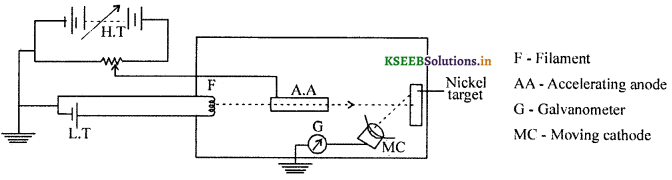This confirm that electron in motion behave as wave.Question 5.
Explain Heisenberg’s uncertainty principle.
Heisenberg’s uncertainty principle states that, it is not possible to measure both the position and the momentum of an electron or any particle exactly at the same time.

There is always some uncertainty (Δx) in the specification of positron and some uncertainty in the linear momentum (Δp).
i. e., Δx. Δp = h/2π = h where Δx ≠ 0 and Δp ≠ 0
If Δx = 0 then Δp should be infinity and if Δp = 0 then Δx = infinity.
The matter wave associated with electron is not extended all over space. Instead, it is a wave packet extending, over some finite region of space. However, a wave packet of finite extension does not have a single wavelength. A wave packet consists of waves spread around someThe matter wave corresponding to a definite momentum of an electron, extends all over space### 2nd PUC Physics Dual Nature of Radiation and Matter List of Formula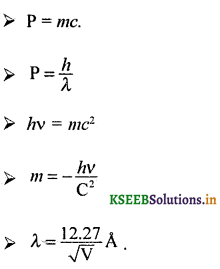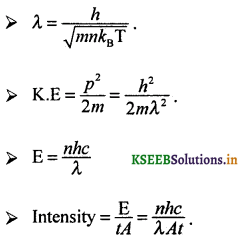### 2nd PUC Physics Dual Nature of Radiation and Matter Numericals and Solutions

Question 1.
A photon has a wavelength of 5000 Å. Calculate its momentum.
Given λ = 5000 Å, p = ?
λ = $$\frac{h}{p}$$Question 2.
Calculate de Broglie wave length of an object of mass 10 kg hurled with a speed of 10 ms-1.
Given m = 10 kg, v = 10 ms-1
We know that, λ = $$\frac{h}{m v}$$
λ = $$\frac{6.626 \times 10^{-34}}{10 \times 10}$$
λ = 6.626 × 10-36 m which is too low to be measured.

Question 3.
The wave length of a photon is 5000 Å. Calculate its energy in electron volts.
Ans.
λ = 5000 Å = 5 × 10-7 m
The energy of a photon is given by E = hv = $$\frac{h c}{\lambda}$$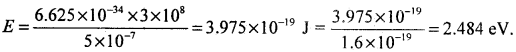Question 4.
Calculate the frequency of light photon of energy 2.24 eV.
Given E = 2.24 eV = 2.24 × 1.6 × 10-19 J, V = ?Question 5.
Light of wavelength 2000 Å can just eject electrons from a metal surface. Calculate the work function of the metal.
Given, λ = 2000 Å = 2 × 107m, w = ?
We know that, w = hv0 = $$\frac{h c}{\lambda}$$Question 6.
Calculate the longest wavelength of incident light on platinum for which photo electrons are just emitted from platinum (work function for platinum 6.2 eV).
Given, w = 6.2 eV = 6.2 × 1.6 × 10-19 J, λmax = ?
w = hv0 = $$\frac{h c}{\lambda_{0}}$$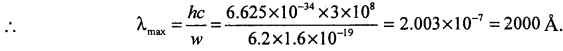Question 7.
The photo electric threshold for a metal surface is 5800 Å. Calculate the kinetic energy of a photoelectron, when a light of wavelength 4500 Å is incident on the metal surface.
Given, λ0 = 5800 Å = 5.8 × 10-7 m
λ = 4500 Å = 4.5 × 10-7m
E = hv =$$\frac{h c}{\lambda}$$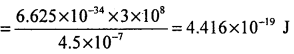Work function of the photo metal is given byFrom Einstein photo electric equation,
E = w + Ek
i.e., Ek = (4.416 – 3.426) × 10-19 = 0.99 × 10-19 J.

Question 8.
Calculate the retarding potential required to stop the photoelectrons emitted from a metal surface of work function 1.2 eV, when light of frequency 6.3 × 1014 Hz is incident on it.
Given w = 1.2eV, v = 6.3 × 1014 HzFrom Einstein’s equation, K.E. of emitted electrons is given by,
Ek = E – w = 2.608 – 1.2eV = 1.408eV.
∴ Stopping potential = 1.408 V.

Question 9.
The work function of copper is 4.57 eV. If radiation of 2500 Å is incident on copper, calculate the maximum velocity of photo electrons. (July 2014)
w = 4.57 eV = 4.57 × 1.6 × 10-19 = 7.3 12 × 10-19 J
Energy of incident photon is
E = hv = $$\frac{h c}{\lambda}$$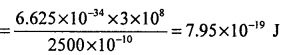According to Einstein’s photo electric equation,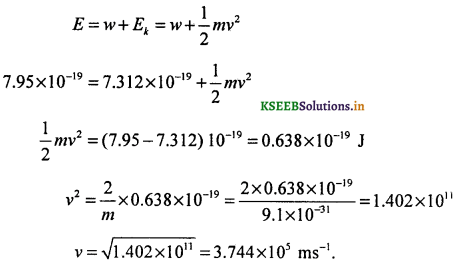Question 10.
A certain sodium lamp radiates 400 W of yellow light of wave length 5896 Å. Calculate the number of photons, emitted by this light in each second (c = 3 × 108 ms-1).
Given : λ = 5.896 × 107 m
Total energy emitted per second E’ = 400 W, No. of photons emitted per second n = ?Question 11.
Calculate the velocity of the photoelectrons emitted when a light of frequency 3 × 1012 Hz is incident on a metal surface of threshold frequency equal to 2 × 1012 Hz.
Given, v = 3 × 1012 Hz, v0 = 2 × 1012Hz.
By photoelectric equation, h v = hv0 + (KE)max
∴ (KE)max = h(v – v0)
= 6.626 × 10-34 × 1012 (3 – 2) = 6.626 × 10-22 J.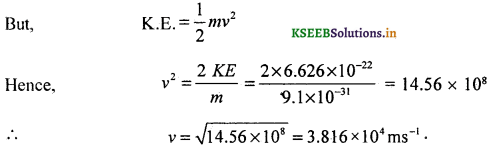Maximum velocity of electron = 3.8 × 104 ms-1.

Question 12.
Ultraviolet radiations of wavelengths 800 Å and 700 Å when allowed to fall on Hydrogen atoms in their ground state, were found to liberate electrons with maximum kinetic energies 1.96 eV and 4.18 eV respectively. Calculate Planck’s constant and work function or ionisation energy of the hydrogen atom.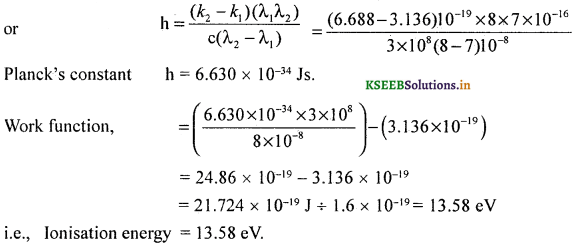Question 13.
A photon of frequency 1.5 × 1015 Hz is incident on a metal surface of work function 1.672 eV. Calculate the stopping potential.
Given : v = 1.5 × 1015 Hz
w = 1.672 eV = 1.672 × 1.6 × 10-19 = 2.675 × 10-19 J.
We know that, E = w + (KE)max
where, E- hv = 6.626 × 10-34 × 1.5 × 1015 = 9.939 × 10-19 J.
∴ (KE)max = (9.939-2.675) × 10-19J = 7.264 × 10-19J ÷ 1.6 × 10-19 = 4.54 eV
∴ Stopping potential = 4.54 V.

Question 14.
Find the (a) maximum frequency and (b) minimum wavelength of X-rays produced by 30 kV electrons.
Using hv = eV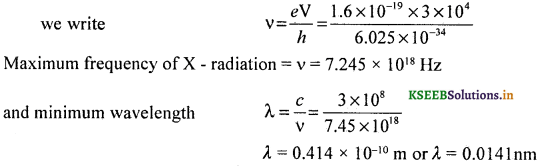Question 15.
The photo electric cut off voltage in a certain experiment is 1.5V. What is the maximum kinetic energy of photoelectrons emitted?
Kinetic energy = 1.5 eV
= 1.6 × 10-19 × 1.5
K.E. = 2.40 × 10-19 J or 1.5eV.

Question 16.
In an experiment on photo electric effect, the slope of cut – off voltage versus frequency, incident light is found to be 4.12 × 10-15 Vs. Calculate the value of Planck’s constant.
Given, slope = 4.12 × 10-15 Vs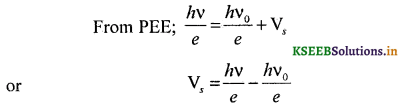Here h/e represents the slope
h/e = 4.12 × 10-15 JsC-1
∴ Planck’s constant h = 4.12 × 10-15 × 1.6 × 10-19 Js = 6.592 × 10-34 Js.

Question 17.
The energy flux of sunlight reaching the surface of Earth is 1.388 × 103 Wm-2 How many photons per square meter are incident on Earth per second? Assume the wavelength of photon at an average of 550nm.Question 18.
Calculate the (i) momentum (ii) speed and (iii) de Broglie wavelength of electron with kinetic energy = 120eVQuestion 19.
An electron and a photon each have a wavelength of 1.00 nm. Find
(i) their momenta
(ii) the energy of the photon and
(iii) K.E. of electron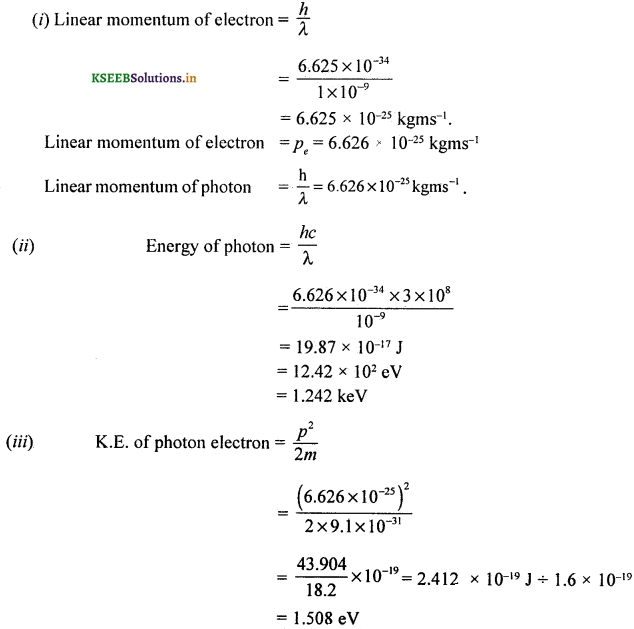Question 20.
(a) For what K.E. of a neutron, will the associated de Broglie wavelength be 1.40 × 10-10m?
(b) Also find the de – Broglie wavelength of a neutron, in thermal equilibrium with matter, having an average kinetic energy of (3/2)kT at 300K